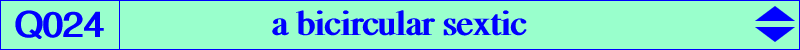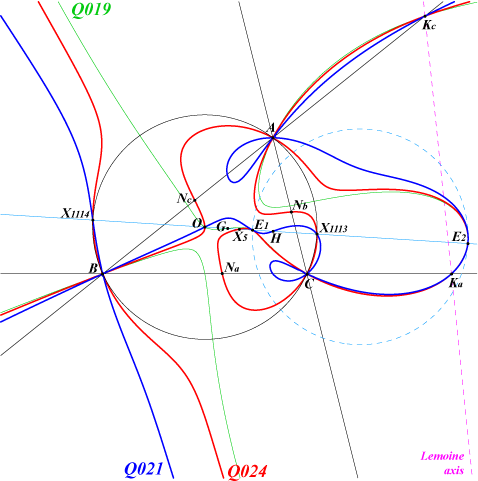too complicated to be written here. Click on the link to download a text file.X(3), X(5), X(1113), X(1114), X(5000), X(5001) points at infinity of the rectangular circum-hyperbola passing through X(140). Na, Nb, Nc : vertices of the cevian triangle of X(5) Ka, Kb, Kc : feet of the Lemoine axis (centers of the apollonian circles) E1, E2 on the figure are X(5001), X(5000) respectively X(5)-circumcevian points (see Q141) anti-points, see Table 77Q024 is a bicircular sextic. It is the locus of P such that P, X(5) and the nine-point center of the circumcevian triangle of P are collinear (together with the line at infinity). Compare Q024 and Q019, Q021. See also Table 55. Q024 meets the Euler line at O, H, X(1113), X(1114) (these two latter points on the circumcircle) and two (not always real) other points E1, E2 on the circle centered at X(468) orthogonal to the circumcircle. These two points also lie on the circum-hyperbola through X(6), X(232), X(250), X(262), X(264), X(325), X(511), X(523), X(842), X(2065) which is the isogonal conjugate of the line passing through the Tarry point X(98) and the focus X(110) of the Kiepert parabola. The nodal tangents at A, B, C are perpendicular. More generally, let X be a center on the Euler line such that OX = k OH (vectors, k real number different of 0) and X' the same center in A'B'C', the circumcevian triangle of P. P, X, X' are collinear if and only if P lies on a bicircular sextic passing through A, B, C (double points with perpendicular nodal tangents if and only X = X(5)), O, X(1113), X(1114), the centers of the Apollonian circles, the cevians of X in ABC, the two points E1, E2. Its has two real asymptotes parallel to those of the rectangular circum-hyperbola passing through Y such that OY = (1-k)/2 OH. This sextic decomposes into the circumcircle and a circular quartic if and only if X = G. This is Q019. When X = O, the locus is the entire plane.